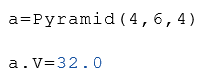# Function Cone

Calculation of the properties of cones

### Instructions

The Cone function calculates several properties of a cone.

The radius and the height are given as arguments.

The function returns a list with the following properties of the cone.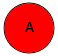A Area of the base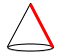L Height of the slope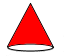S Surface without a base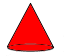Sg Surface with base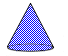V Volume

### Syntax

Calculation of the properties from the radius $$r$$ and the height $$h$$.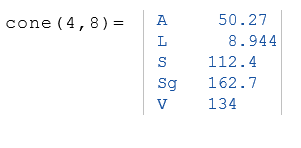In the example below, only the volume $$V$$ of the cone is displayed.